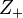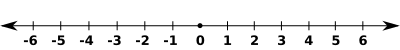# Positive number facts for kids

Kids Encyclopedia Facts

A positive number, also known as a positive integer, is a number that is bigger than zero. The set of all positive integers is written as${Z}_+$.

A positive number can be written with a "+" symbol in front of it, or just as a number. For example, the numbers "+3" and "3" stand for the same numbers.

Positive numbers are written to the right of zero on a number line:In the above line, the positive numbers 1, 2, 3, 4, 5 and 6 are shown.

## Related pagesPositive number Facts for Kids. Kiddle Encyclopedia.